# More Foldable!

Flip your foldable over to the backside (the only part left blank) and add these titles to each box: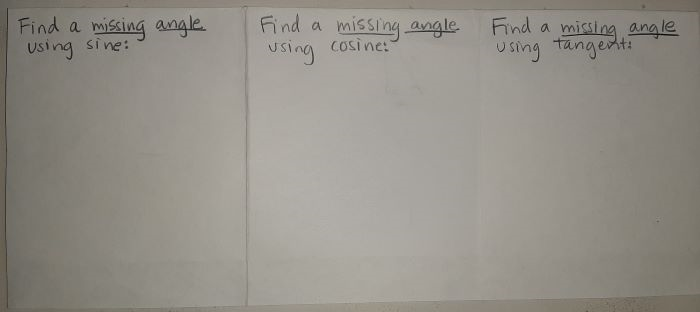next, draw this example triangle and set up the sin ratio: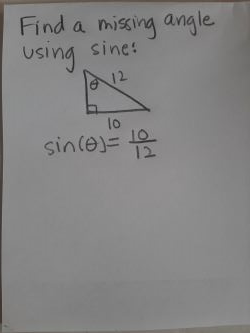and then solve for theta using inverse sin or acrsin: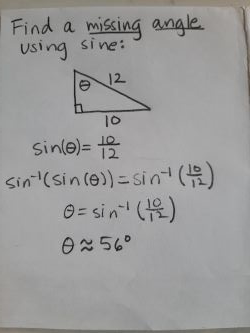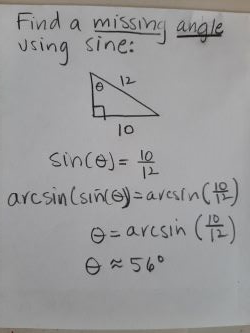then draw this example triangle and set up the cos ratio:

﻿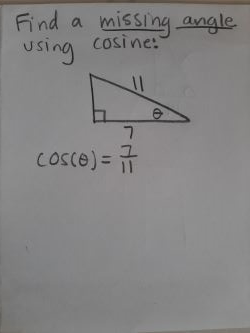and solve for theta using inverse cos or arccos: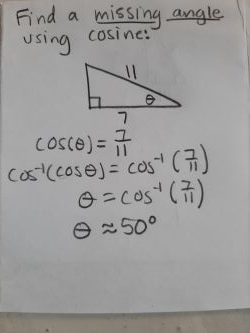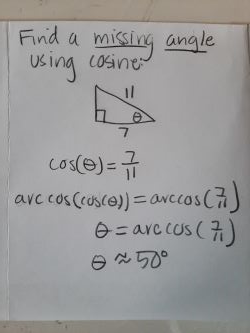Lastly, let's do the same for tangent, start with the example triangle and set up the tangent ratio: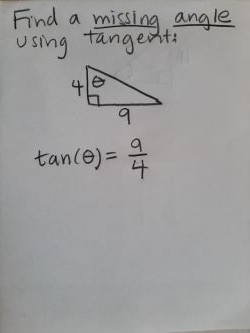then solve for theta using inverse tan or arctan: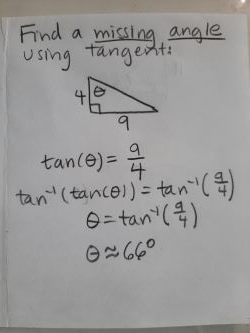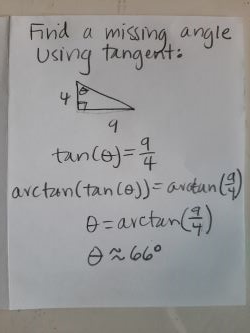When you're all done it should look like this: